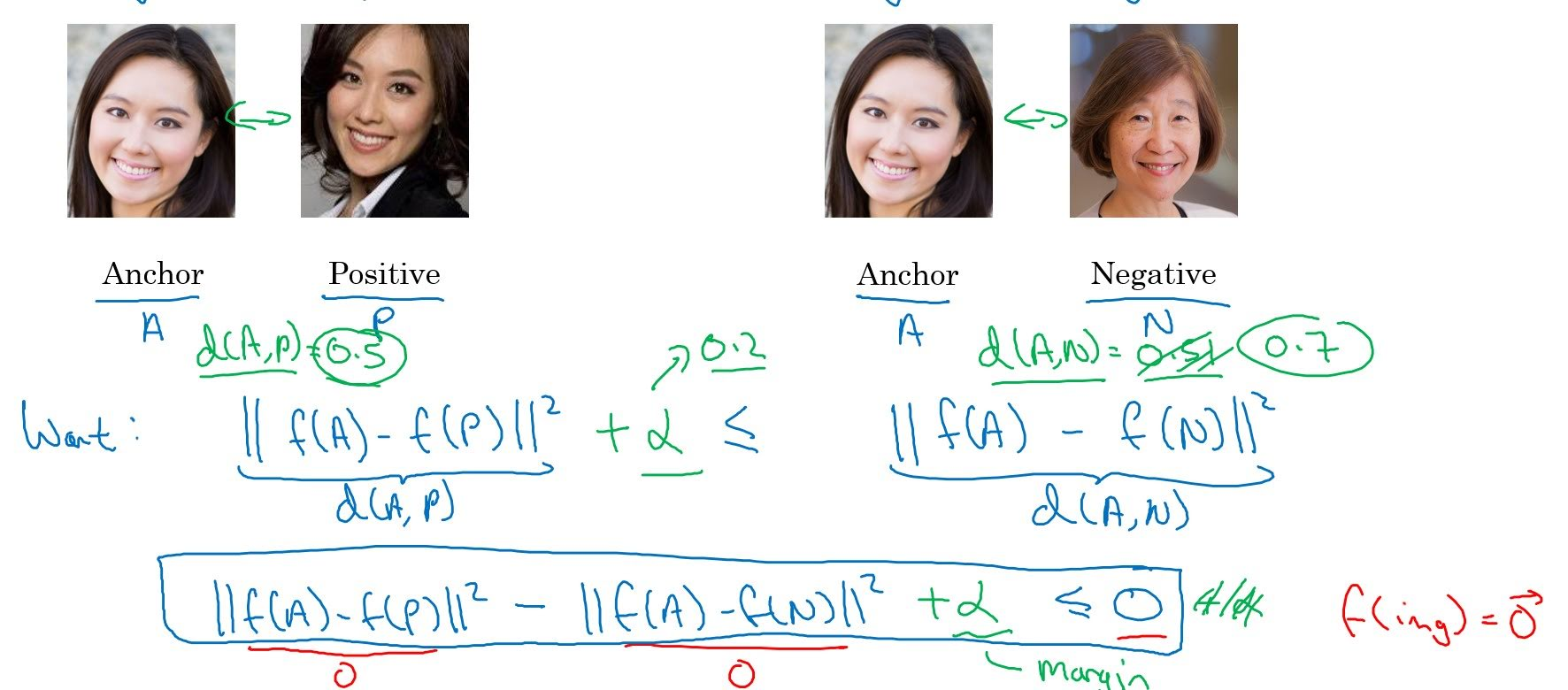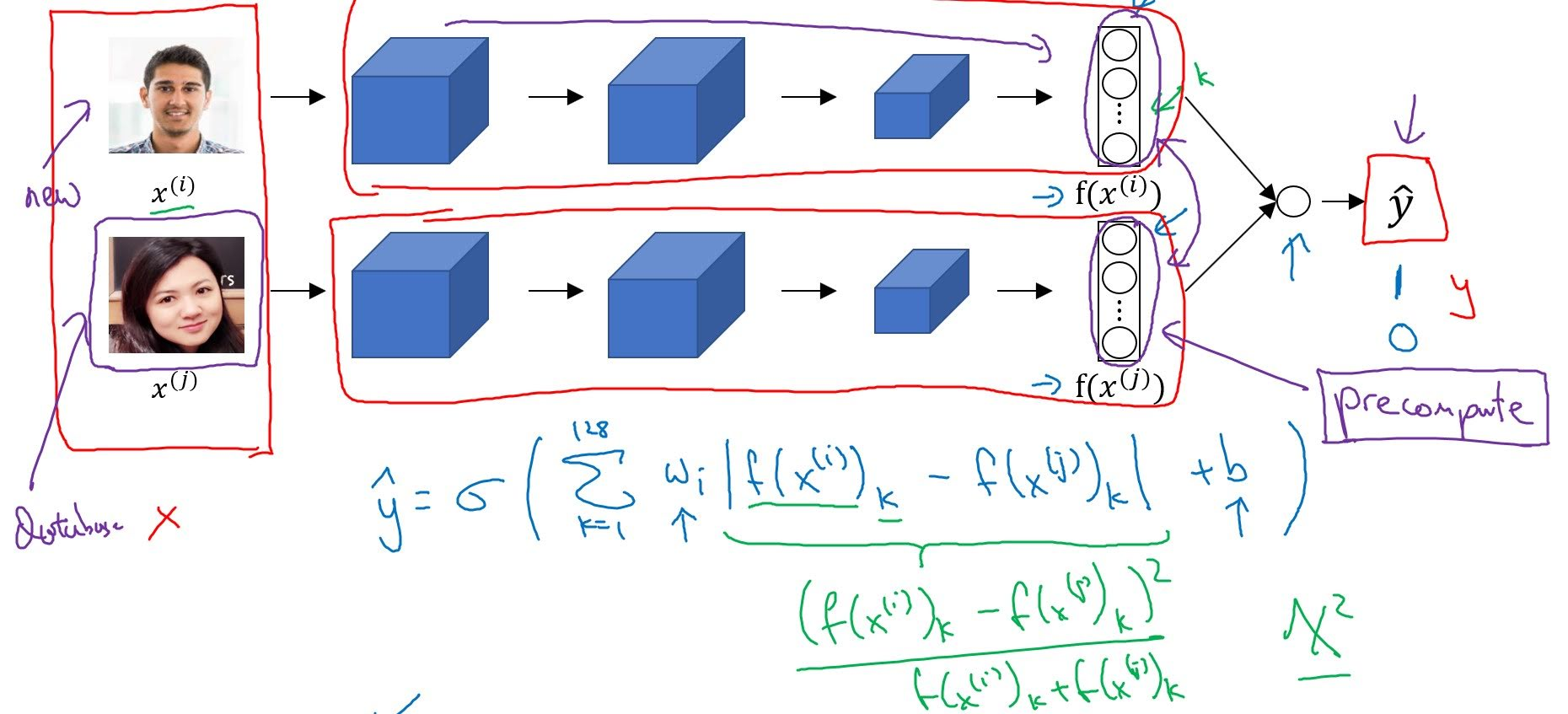# Face Recognition

Categories:

Updated:

https://ieeexplore.ieee.org/document/6909616
https://arxiv.org/abs/1503.03832

# Face Verification vs Face Recognition

Verification: With input face image and name of a person, decide whether they are correct matches

Recognition: With input face image, decide whether that person is in our database.

# One-shot Learning

There are two ways to do face recognition.

1. With input face image, do multiclass classification.
2. With face images, learn a ‘similarity function’. In particular, you want a neural network to learn a function which going to denote d, which inputs two images and outputs the degree of difference between the two images. Using this similarity function, decide if the input face image is similar to one of the face images in our database.

In practice, the number of images of each person is too small to train a multiclass classification CNN. We need to use one-shot learning, which is a learning method that aims to learn information about object categories from one, or only a few, training images. Therefore we use second method.

# Siamese Network

Siamese network encodes an image into a vector.

## Goal of Learning

We want to learn a similarity function, so our goal is as follows;

• If $x^{(i)}, x^{(j)}$ are the same person, make $||f(x^{(i)})-f(x^{(j)})||^2$ small.
• If $x^{(i)}, x^{(j)}$ are different persons, make $||f(x^{(i)})-f(x^{(j)})||^2$ large.

There are two ways to achieve this goal.

### 1. Using Triplet Loss

In the terminology of the triplet loss, what you’re going do is always look at one anchor image ‘A’, a positive image ‘P’(different image of a same person) and negative image ‘N’(image of a different person). You want the distance between the anchor and the positive image to be small, and distance between the anchor and the negative image to be far.In formula, our objective is this;

where $d(A,X)=||f(A)-f(X)||^2$

$\alpha$ is for margin. If there is no $\alpha$, CNN can just learn to output 0s to satisfy the objective.

Our loss function will be as follows;

We use max because we only have to satisfy our objective $d(A,P)-d(A,N) + \alpha \leq 0$. If this condition is satisfied, our loss will be always 0.

Then our cost function would be;

In concolusion, with input images, train a convnet to encode each image and find a similarity function of a pair of images. We then use our cost function $J$ and gradient descent to optimize parameters.

Note that during training, if A, P, N are chosen randomly, our objective is easily satisfied. So, choose triplets that’re “hard” to train on.

### 2. Via Binary ClassificationWe take two images, and pass each of them into same convnet to output encodings of each image $f(x^{(i)}), f(x^{(j)})$. Let’s say our encoding vector has 128 values.

With encodings, do the following to output binary prediction(1 if two images are from same person, 0 if not).

Instead of $|f(x^{(i)})_k-f(x^{(j)})_k|$, you can also use chi-squared similarity $\frac{(f(x^{(i)})_k-f(x^{(j)})_k)^2}{f(x^{(i)})_k+f(x^{(j)})_k}$.

In practice, we pre-encode images in database in advance, and when inputted an new image, encode only that image, then with these two encodings output final prediction.

Categories: